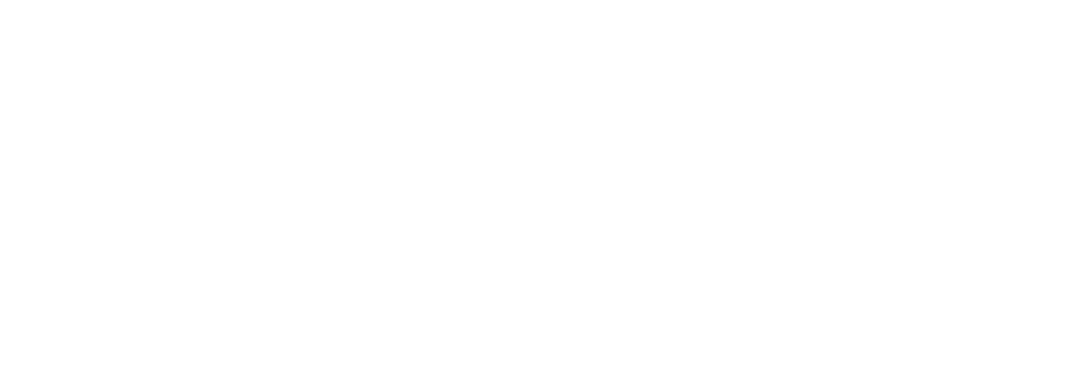1. 不同子集的交集等于空集。

2.k 个子集的并集为 S。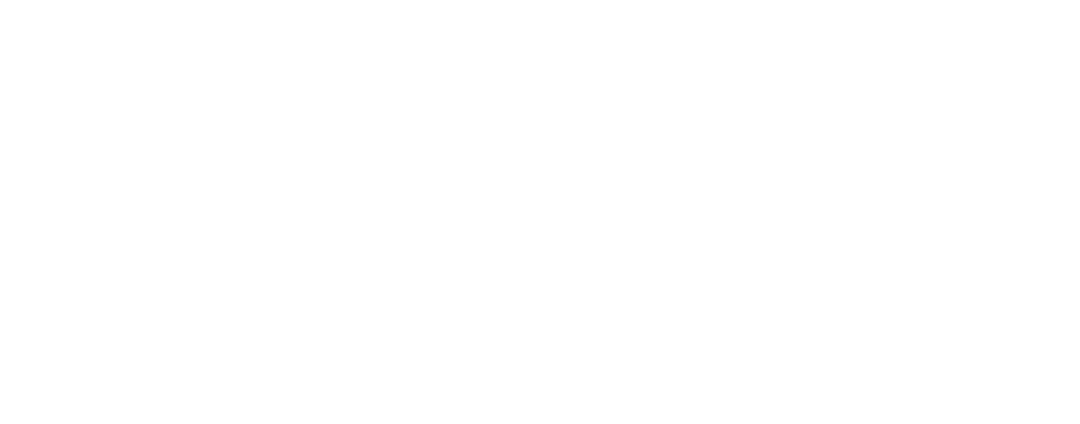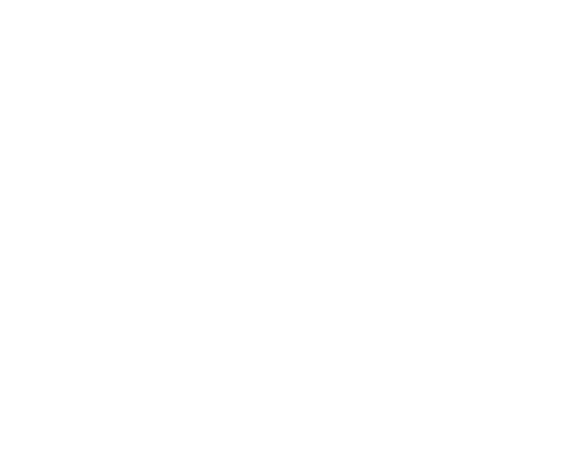k=2 数据分区的质心演示示例。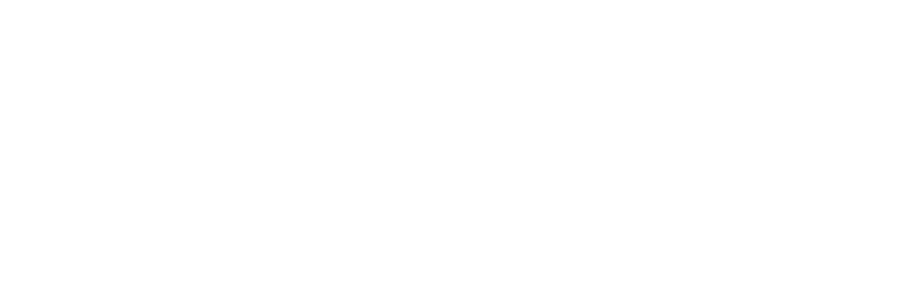``@add_metaclass(ABCMeta)class PartitionalClusteringObjectiveFunction(ObjectiveFunction): def __init__(self, dim, n_clusters, data): super(PartitionalClusteringObjectiveFunction, self) .__init__('PartitionalClusteringObjectiveFunction', dim, 0.0, 1.0) self.n_clusters = n_clusters self.centroids = {} self.data = data def decode(self, x): centroids = x.reshape(self.n_clusters, self.dim) self.centroids = dict(enumerate(centroids)) @abstractmethod def evaluate(self, x): passclass SumOfSquaredErrors(PartitionalClusteringObjectiveFunction): def __init__(self, dim, n_clusters, data): super(SumOfSquaredErrors, self).__init__(dim, n_clusters, data) self.name = 'SumOfSquaredErrors' def evaluate(self, x): self.decode(x) clusters = {key: [] for key in self.centroids.keys()} for instance in self.data: distances = [np.linalg.norm(self.centroids[idx] - instance) for idx in self.centroids] clusters[np.argmin(distances)].append(instance) sum_of_squared_errors = 0.0 for idx in self.centroids: distances = [np.linalg.norm(self.centroids[idx] - instance) for instance in clusters[idx]] sum_of_squared_errors += sum(np.power(distances, 2)) return sum_of_squared_errors``

``import matplotlib.pyplot as pltfrom abc import ABCfrom objection_function import SumOfSquaredErrorsfrom sklearn.datasets import load_irisfrom sklearn.preprocessing import MinMaxScalerdata = MinMaxScaler().fit_transform(load_iris()['data'][:, [1,3]])plt.figure(figsize=(9,8))plt.scatter(data[:,0], data[:,1], s=50, edgecolor='w', alpha=0.5)plt.title('Original Data')``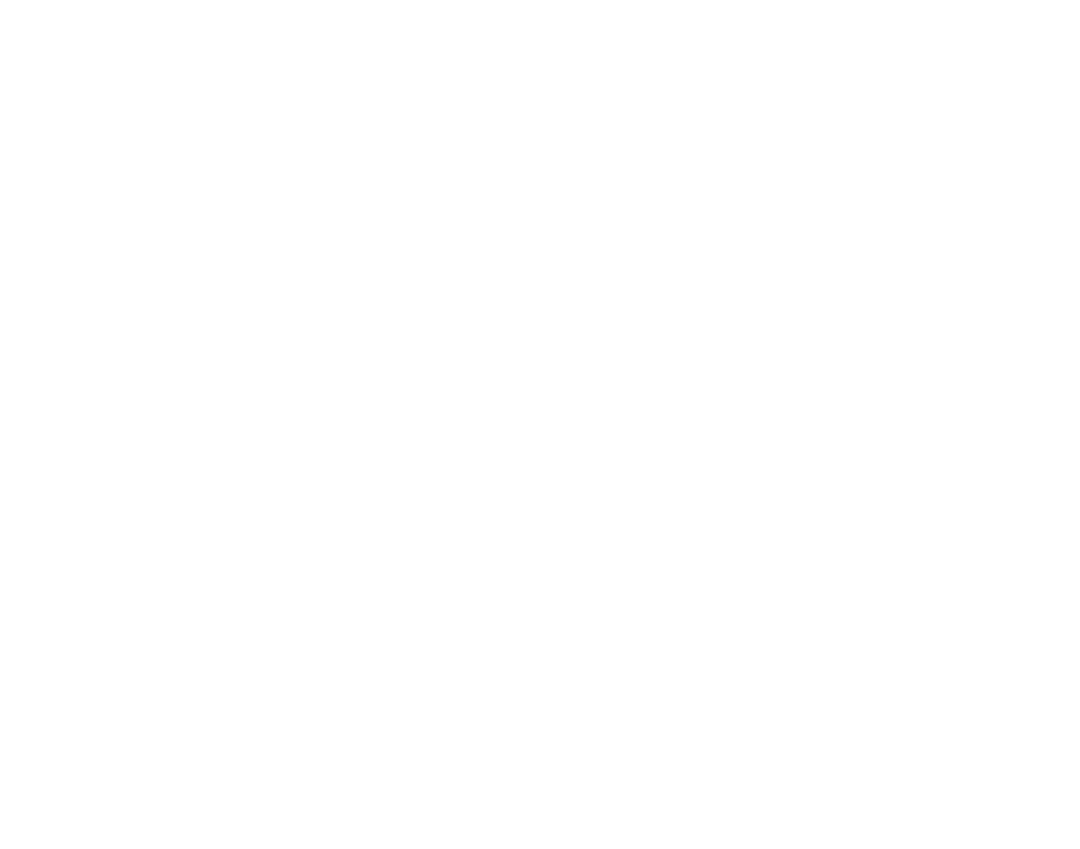``colors = ['r', 'g', 'y']target = load_iris()['target']plt.figure(figsize=(9,8))for instance, tgt in zip(data, target): plt.scatter(instance, instance, s=50, edgecolor='w', alpha=0.5, color=colors[tgt])plt.title('Original Groups')``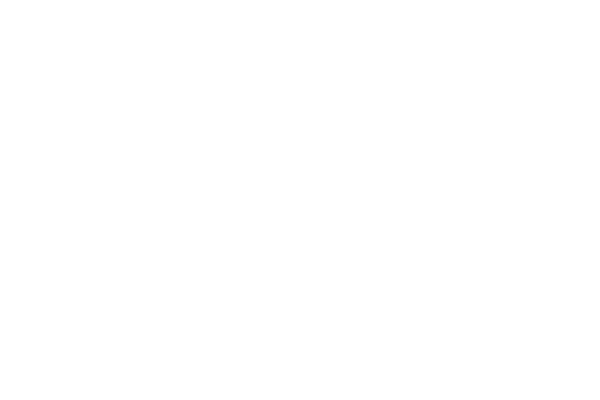``objective_function = SumOfSquaredErrors(dim=6, n_clusters=3, data=data)optimizer = ABC(obj_function=objective_function, colony_size=30, n_iter=300, max_trials=100)optimizer.optimize()def decode_centroids(centroids, n_clusters, data): return centroids.reshape(n_clusters, data.shape)centroids = dict(enumerate(decode_centroids(optimizer.optimal_solution.pos, n_clusters=3, data=data)))def assign_centroid(centroids, point): distances = [np.linalg.norm(point - centroids[idx]) for idx in centroids] return np.argmin(distances)custom_tgt = []for instance in data: custom_tgt.append(assign_centroid(centroids, instance))colors = ['r', 'g', 'y']plt.figure(figsize=(9,8))for instance, tgt in zip(data, custom_tgt): plt.scatter(instance, instance, s=50, edgecolor='w', alpha=0.5, color=colors[tgt])for centroid in centroids: plt.scatter(centroids[centroid], centroids[centroid], color='k', marker='x', lw=5, s=500)plt.title('Partitioned Data found by ABC')``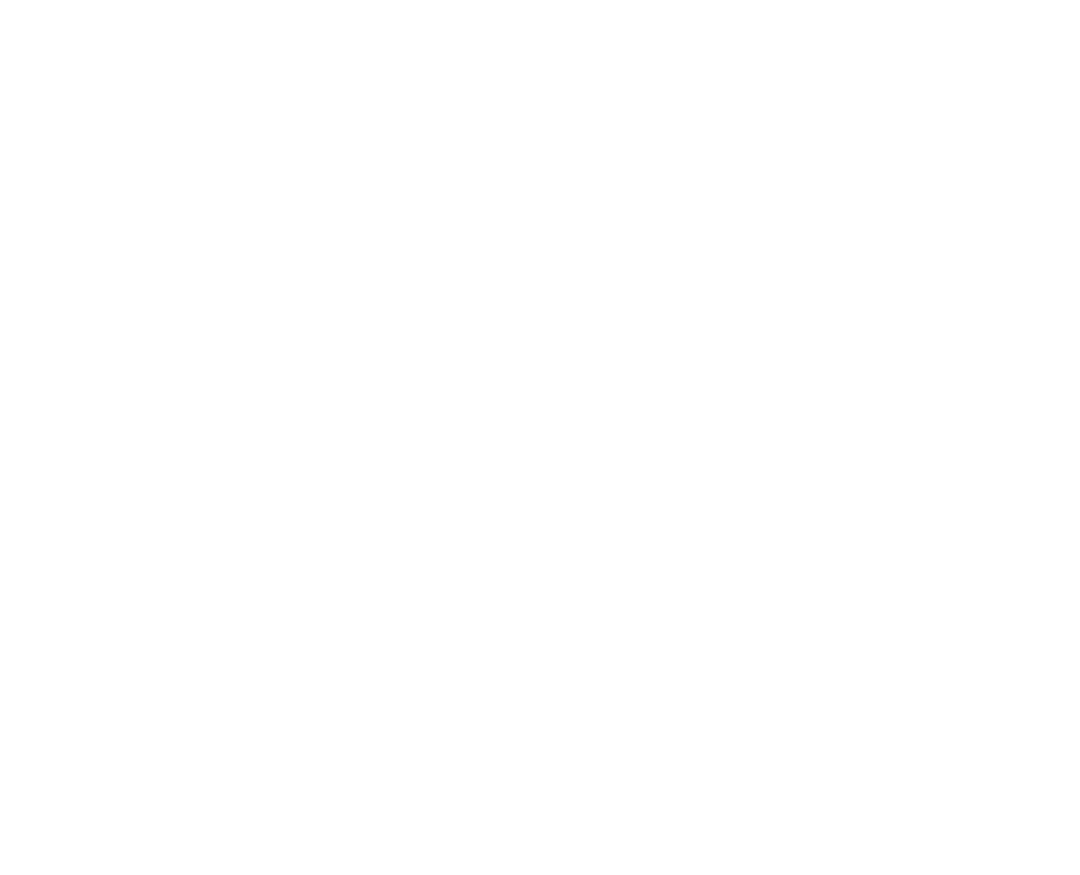ABC 算法生成的分区

``itr = range(len(optimizer.optimality_tracking))val = optimizer.optimality_trackingplt.figure(figsize=(10, 9))plt.plot(itr, val)plt.title('Sum of Squared Errors')plt.ylabel('Fitness')plt.xlabel('Iteration')``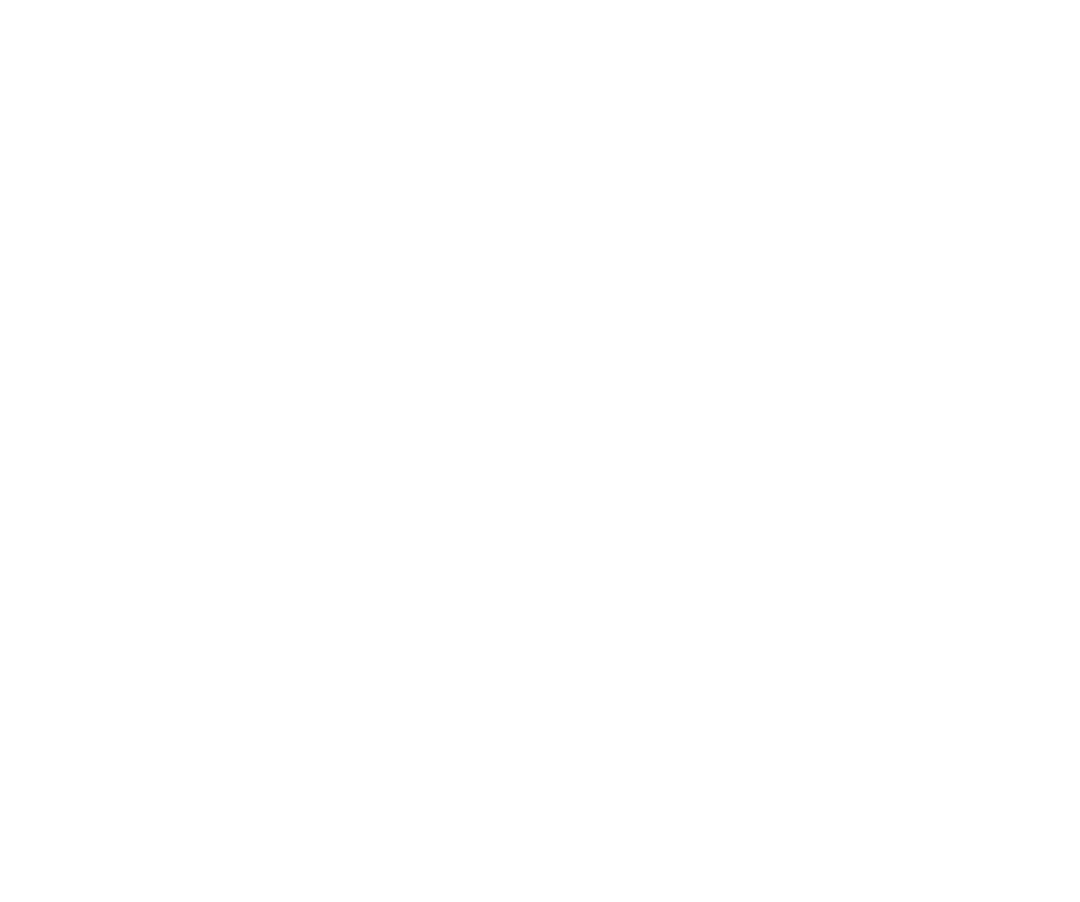• A novel clustering approach: Artificial Bee Colony (ABC) algorithm—Dervis Karaboga, Celal Ozturk

• A Clustering Approach Using Cooperative Artificial Bee Colony Algorithm—Wenping Zou, Yunlong Zhu, Hanning Chen, and Xin Sui

• A Review on Artificial Bee Colony Algorithms and Their Applications to Data Clustering—Ajit Kumar , Dharmender Kumar , S. K. Jarial

• A two-step artificial bee colony algorithm for clustering—Yugal Kumar, G. Sahoo